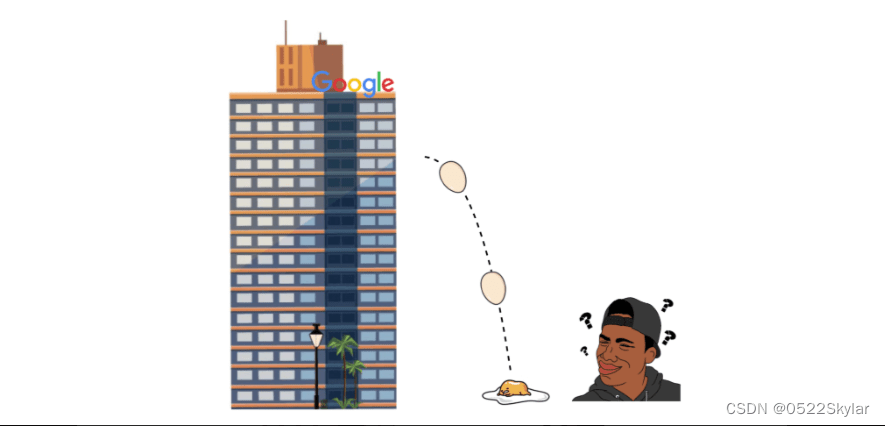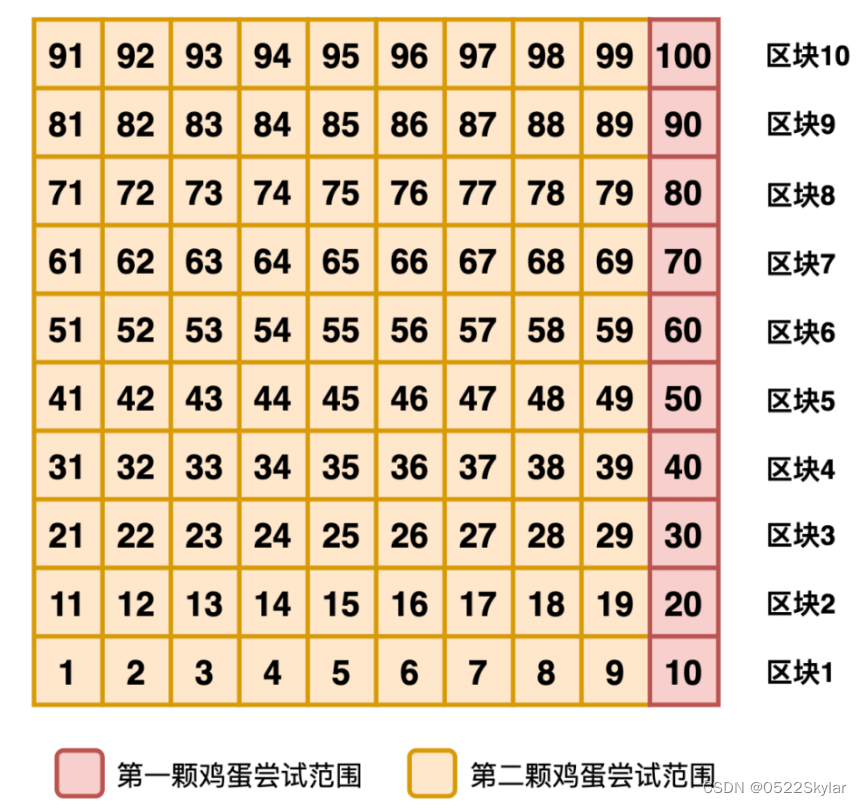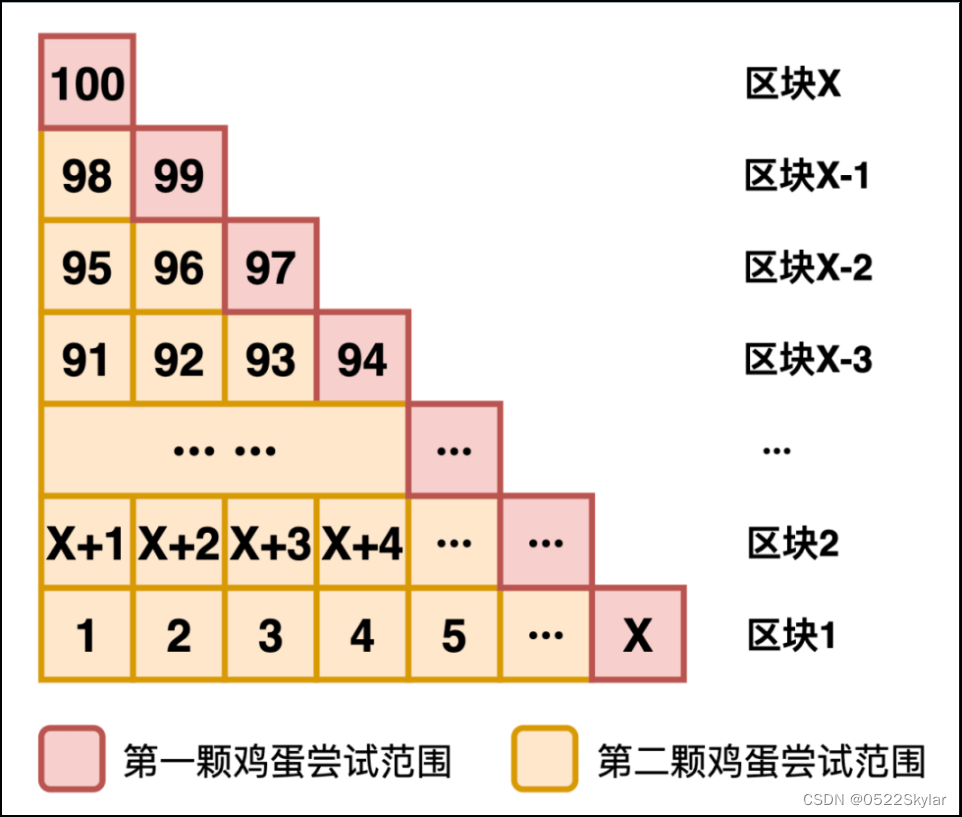# 谷歌面试中的扔鸡蛋问题解析

1. 没有摔碎的鸡蛋可以重复使用；

2. 每颗鸡蛋的坚硬程度都是相同的。### 解法1：简单粗暴

``````// 假设arr表示100层楼,每层楼鸡蛋会不会碎,如果arr[i] === 1 表示i层楼的鸡蛋会碎,arr[i] === 0表示第i层楼的鸡蛋不会碎

// 简单暴力
const throwEggs1 = (arr) => {
for(let i = 1; i <= 100; i++) {
if (arr[i] === 1) {
return i
}
}
}
``````

### 解法2：常规二分

``````// 常规二分

const throwEggs2 = (arr) => {
let left = 1
let right = 100
let mid = 0
while(left <= right) {
mid = Math.floor(left + right) / 2
if (arr[mid] === 0) {
left = mid + 1
} else {
right = mid - 1
}
}
return mid
}
``````

### 解法3：均衡切割``````// 均衡切割
const throwEggs3 = (arr) => {
let count = 0
// 第一个鸡蛋
for (let i = 1; i < 10; i++) {
if (arr[i * 10] === 1) {
count = i * 10
break
}
}
// 第二个鸡蛋
for(let i = count - 1; i >= count - 10; i--) {
if (arr[i] !== 1) {
return i
}
}
}
``````

### 解法4：微妙平衡

X+（X-1）+（X-2）+···+3+2+1≥100X(X+1)/2≥100

······``````// 假设arr表示100层楼,每层楼鸡蛋会不会碎,如果arr[i] === 1 表示i层楼的鸡蛋会碎,arr[i] === 0表示第i层楼的鸡蛋不会碎

// 暴力
const throwEggs1 = (arr) => {
for(let i = 1; i <= 100; i++) {
if (arr[i] === 1) {
return i
}
}
}

// 常规二分

const throwEggs2 = (arr) => {
let left = 1
let right = 100
let mid = 0
while(left <= right) {
mid = Math.floor(left + right) / 2
if (arr[mid] === 0) {
left = mid + 1
} else {
right = mid - 1
}
}
return mid
}

// 均衡切割
const throwEggs3 = (arr) => {
let count = 0
// 第一个鸡蛋
for (let i = 1; i < 10; i++) {
if (arr[i * 10] === 1) {
count = i * 10
break
}
}
// 第二个鸡蛋
for(let i = count - 1; i >= count - 10; i--) {
if (arr[i] !== 1) {
return i
}
}
}

// 微妙平衡
const throwEggs4 = (arr) => {
let block = 10
let count = 0
// block(block + 1) / 2 >= 100
while(block * block + block < 100 * 2) {
block++
}
// 第一个鸡蛋的尝试
let temp = block // 每层区块的最后一个
while(temp <= 100) {
if (arr[temp] === 1) {
count = temp
break
}
--block
temp += block
}

// 第二个鸡蛋的尝试
for(let i = count - 1; i >= count - block; i--) {
if (arr[i] === 0) {
return i
}
}
}
``````